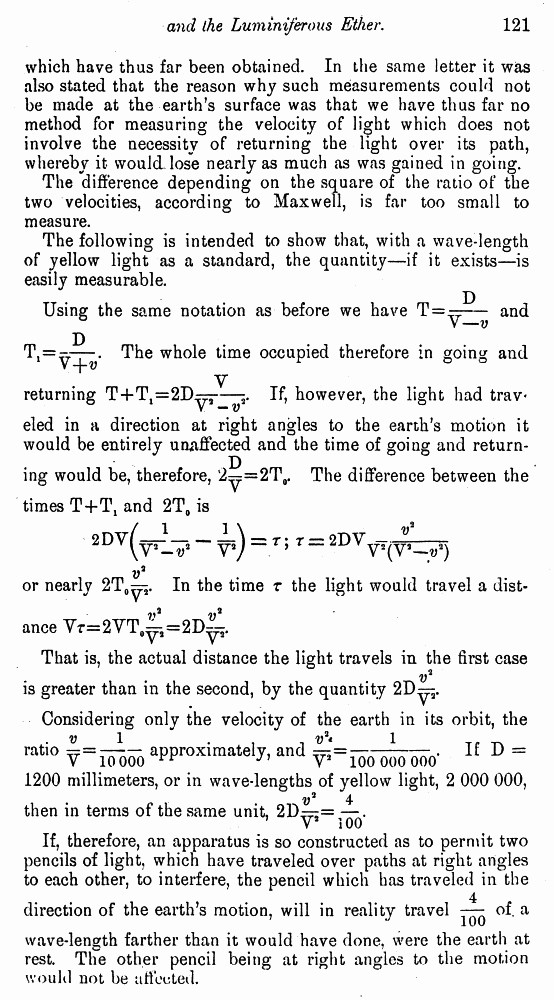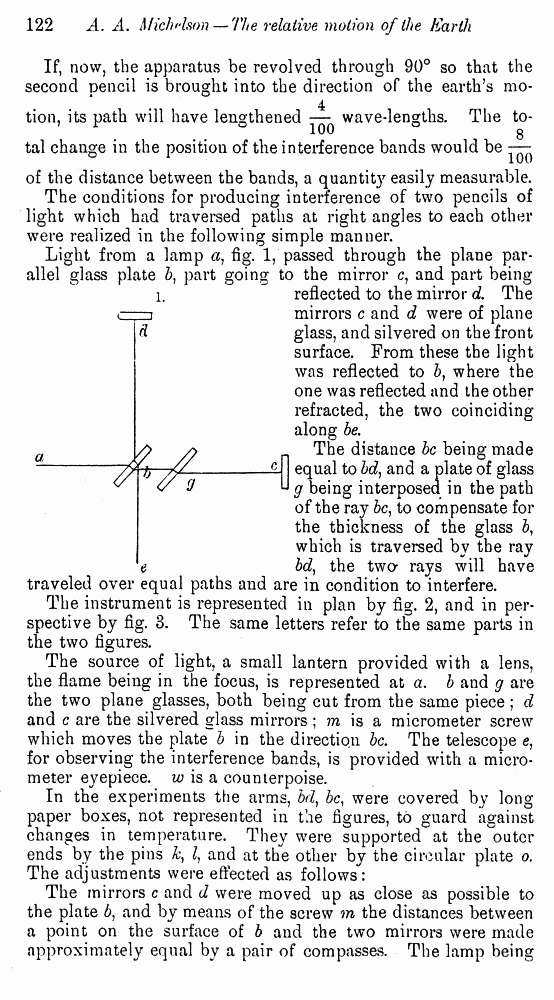# Albert A. Michelson. The Relative Motion of the Earth and the Luminiferous Ether // American Journal of Science, 1881, 22: Page 121

В начало   Другие форматы   <<<     Страница 2   >>>1  2 3  4  5  6  7  8  9  10 which have thus far been obtained. In the same letter it was also stated that the reason why such measurements could not be made at the earth’s surface was that we have thus far no method for measuring the velocity of light which does not involve the necessity of returning the light over its path, whereby it would lose nearly as much as was gained in going. The difference depending on the square of the ratio of the two velocities, according to Maxwell, is far too small to measure. The following is intended to show that, with a wave-length of yellow light as a standard, the quantity—if it exists—is easily measurable. Using the same notation as before we haveand The whole time occupied therefore in going and returningIf, however, the light had trav eled in a direction at right angles to the earth’s motion it would be entirely unaffectedand the time of going and returning would be, therefore,The difference between the times T+T1 and 2T0 is or nearlyIn the timethe light would travel a dist ance That is, the actual distance the light travels in the first case is greater than in the second, by the quantity Considering only the velocity of the earth in its orbit, the ratioapproximately, andIf D = 1200 millimeters, or in wave-lengths of yellow light, 2 000 000, then in terms of the same unit, If, therefore, an apparatus is so constructed as to permit two pencils of light, which have traveled over paths at right angles to each other, to interfere, the pencil which has traveled in the direction of the earth’s motion, will in reality travelof. a wave-length farther than it would have done, were the earth at rest. The other pencil being at right angles to the motion would not be affected. If, now, the apparatus be revolved through 90° so that the second pencil is brought into the direction of the earth’s motion, its path will have lengthenedwave-lengths. The total change in the position of the interference bands would be of the distance between the bands, a quantit}r easily measurable. The conditions for producing interference of two pencils of light which had traversed paths at right angles to each other were realized in the following simple manner. Light from a lamp a) fig. 1, passed through the plane parallel glass plate 5, part going to the mirror c, and part being- reflected to the mirror d. The mirrors c and d were of plane glass, and silvered on the front surface. From these the light was reflected to b, where the one was reflected and the other refracted, the two coinciding along be. The distance be being made equal to bd, and a plate of glass g being interposed in the path of the ray be, to compensate for the thickness of the glass b, which is traversed by the ray bd, the two* rays will have traveled over equal paths and are in condition to interfere. The instrument is represented in plan by fig. 2, and in perspective by fig. 3. The same letters refer to the same parts in the two figures. The source of light, a small lantern provided with a lens, the flame being in the focus, is represented at a. b and g are the two plane glasses, both being cut from the same piece ; d and c are the silvered glass mirrors ; m is a micrometer screw which moves the plate b in the direction be. The telescope e, for observing the interference bands, is provided with a micrometer eyepiece, w is a counterpoise. In the experiments the arms, bd) be, were covered by long paper boxes, not represented in the figures, to guard against changes in temperature. They were supported at the '"outer ends by the pins h, I, and at the other by the circular plate o. The adjustments were effected as follows: The mirrors c and d were moved up as close as possible to the plate 6, and by means of the screw m the distances between a point on the surface of b and the two mirrors were made approximately equal by a pair of compasses. The lamp being